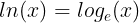# ln(x): calculation and implementation in different programming languages

The natural logarithm of a number is that number’s logarithm to the base of e (a mathematical constant). Shown as a mathematical statement:The natural logarithm of a number is that number’s logarithm to the base of e

To show this better, we could also use:

## Mani Ebrahimi

Shahid Ezheie 1 Highschool student, Iran